# How to chart a running total

Data Pipeline

A running total is a common metric to gain insight into how an amount has changed over time. This process can be easily accomplished in Chartio by utilizing the Data Pipeline to transform your data.

First, open your existing chart or create a new chart if you do not have an existing chart from to work from. At a minimum, you’ll need to include the measure for which you’d like to keep a running total and at least one dimension that will be used to group the running total. For your dimension, we recommend using a date with a time bucket of your choice to best visualize the data you’re interested in seeing such as month or year.

In this example, we’ll be using our SaaS Company Demo Data Data Source. For this chart, we’re using the “Total sum of Amount” for the measure, and the “Month of Payment Date” will be used as our grouping dimension. Once you’ve dragged and dropped your selections, click Run Query to populate the chart.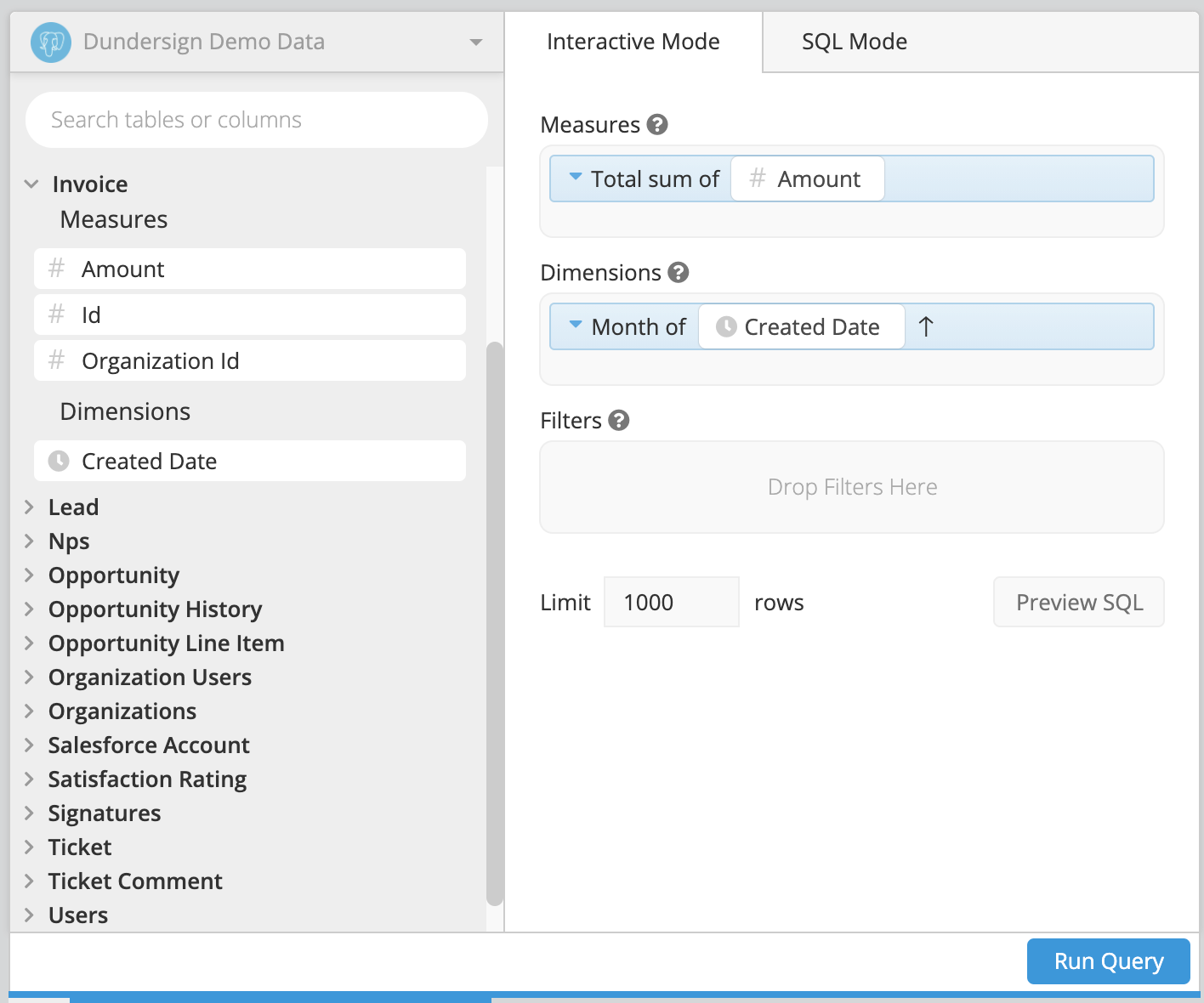You’ll then need to edit the query results using the Data Pipeline. Click + Add Transformation below your Dataset and choose Edit Column or Add Column (if you’d like to keep the original data as part of the chart). Here, we’ll choose Add Column.

Give your new column a name, select Running total from the Formula Type dropdown, and select the column you’d like to be used as the input column for the running total. Click Apply & Close once completed.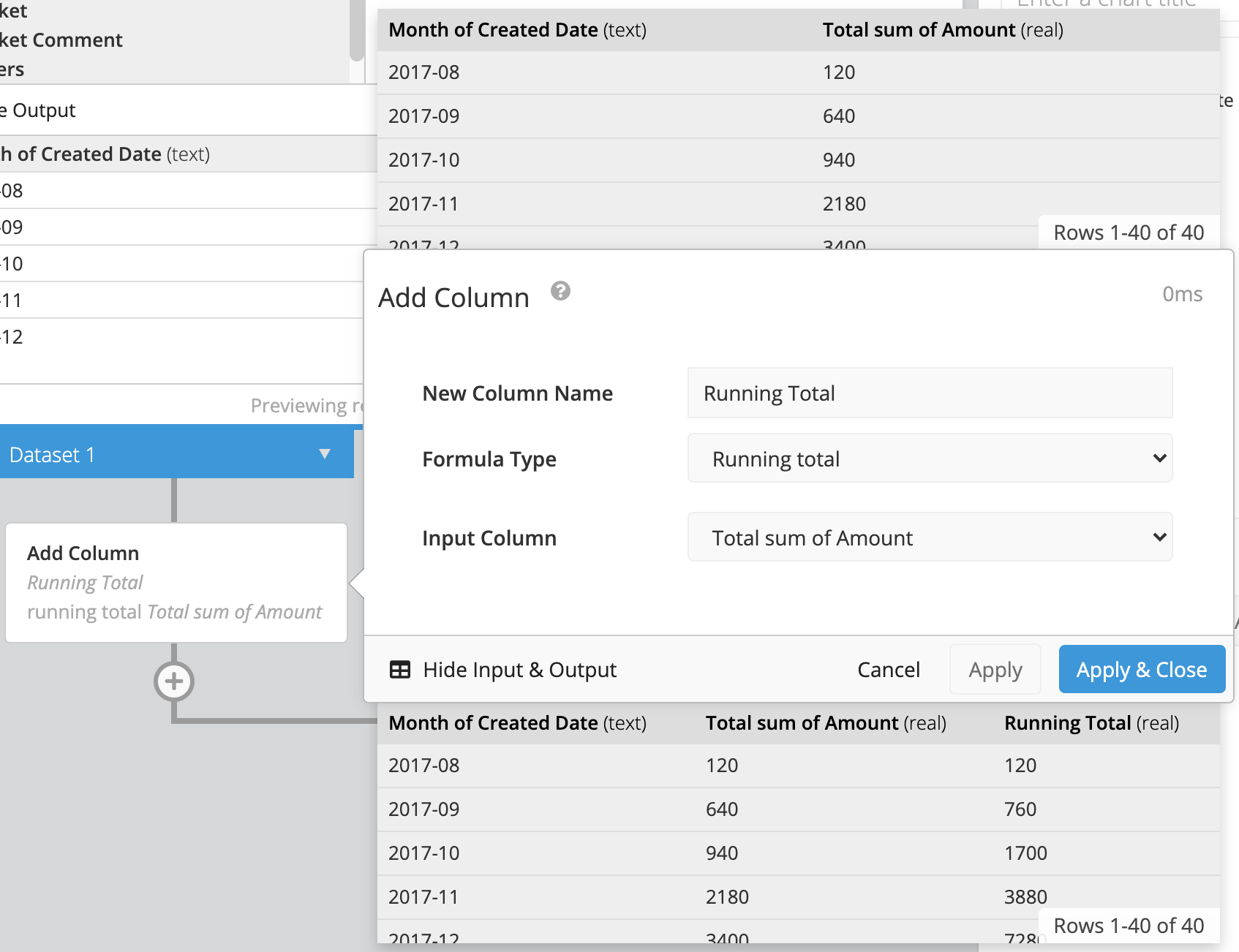In the chart display section, choose the Line chart icon to see more clearly how the total has changed over time.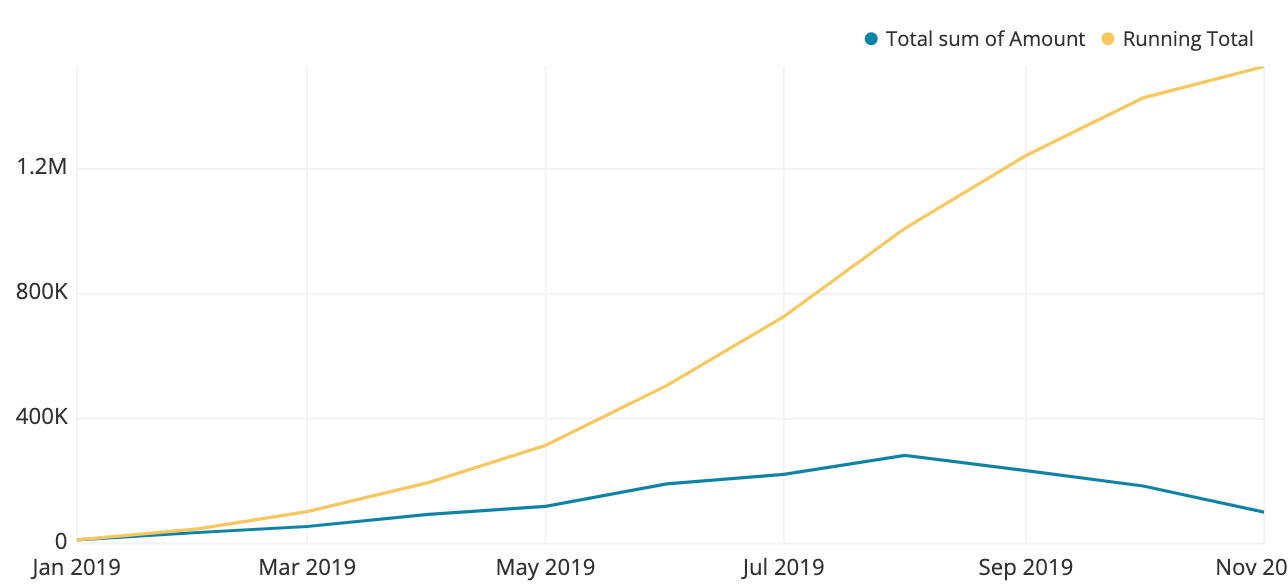## Running total by group

You can also create a running total for groups using SQLite Window Functions.

For example, we’d now like to see the amount we’ve made over time but now, grouped by plan type. To do this, we’ll need to utilize Custom formulas in our Add Column or Edit Column Pipeline steps.

You’ll want to utilize the following statement which calculates the sum of Column1 for the groups in Column2 following the order of rows in Column3.

``````sum("Column1") over (partition by "Column2" order by "Column3")
``````

In this example, we used the following statement to get the running total of the “Total sum of Amount” for each type of plan “Title”, ordered by the “Month of Payment Date”.

``````sum("Total sum of Amount") over (partition by "Title" order by "Month of Payment Date")
``````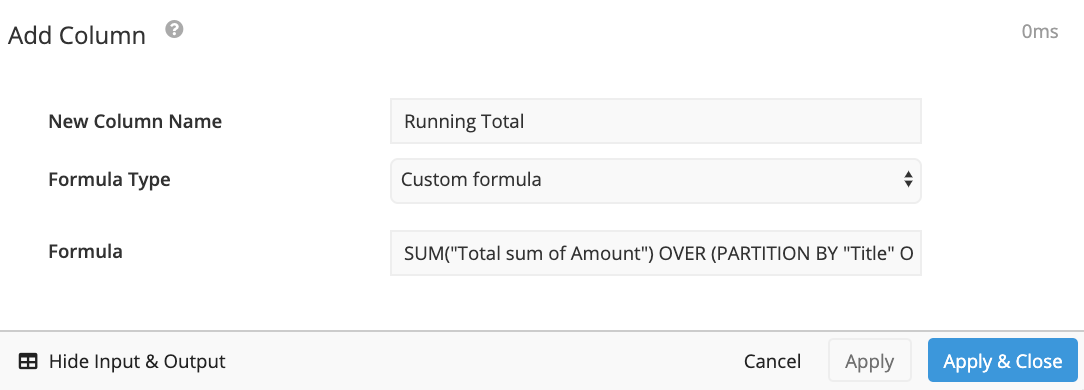We can now see the running total in our table below. As we’ve added a partition by clause, the running total starts over for every new, unique value in the “Title” column. This same formula can be used in a number of ways such as calculating the running total by month.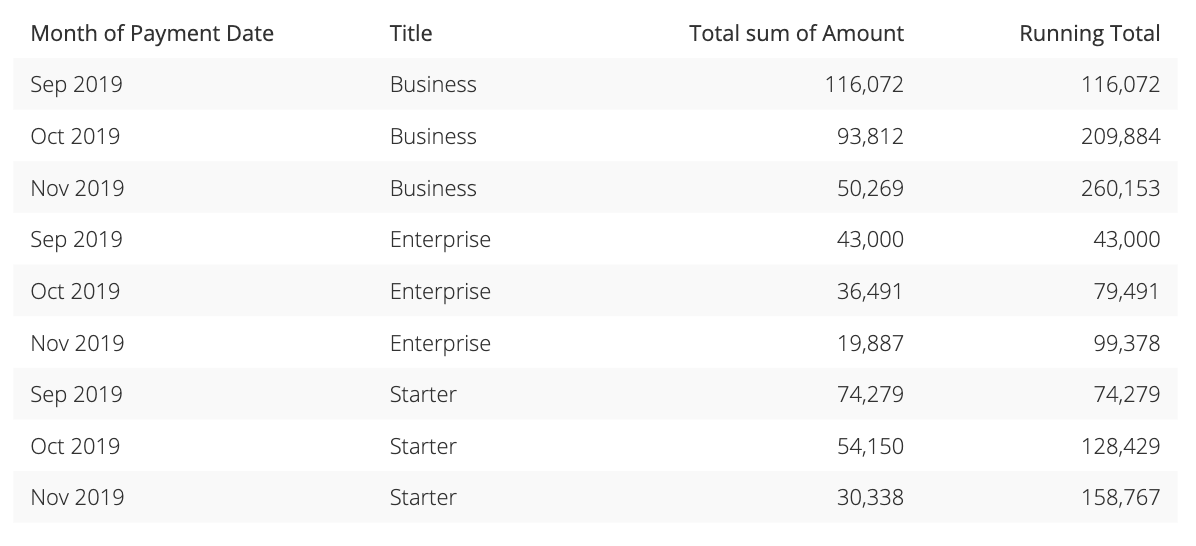See more